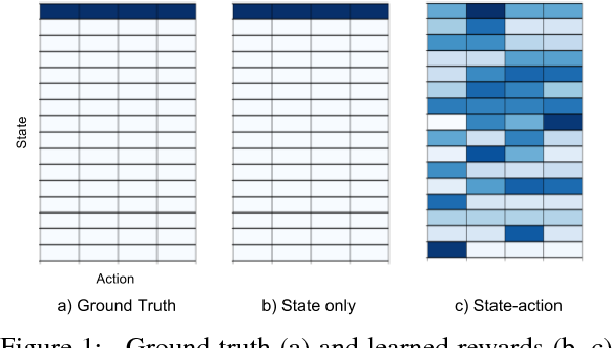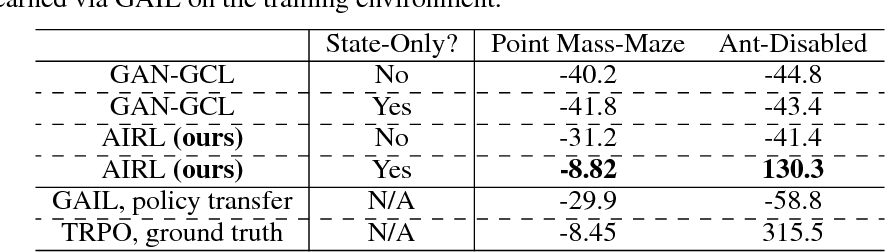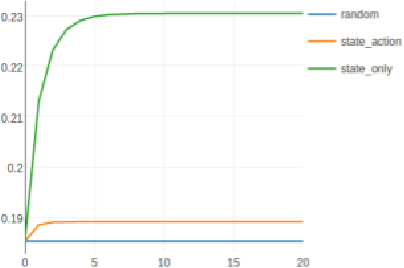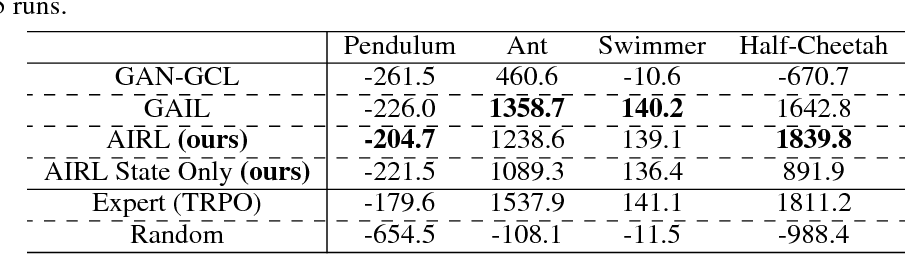Reinforcement learning provides a powerful and general framework for decision making and control, but its application in practice is often hindered by the need for extensive feature and reward engineering. Deep reinforcement learning methods can remove the need for explicit engineering of policy or value features, but still require a manually specified reward function. Inverse reinforcement learning holds the promise of automatic reward acquisition, but has proven exceptionally difficult to apply to large, high-dimensional problems with unknown dynamics. In this work, we propose adverserial inverse reinforcement learning (AIRL), a practical and scalable inverse reinforcement learning algorithm based on an adversarial reward learning formulation. We demonstrate that AIRL is able to recover reward functions that are robust to changes in dynamics, enabling us to learn policies even under significant variation in the environment seen during training. Our experiments show that AIRL greatly outperforms prior methods in these transfer settings.

Click to Read Paper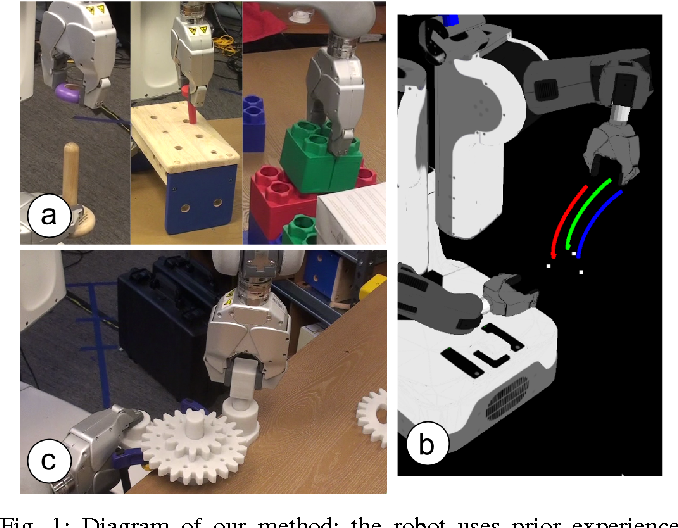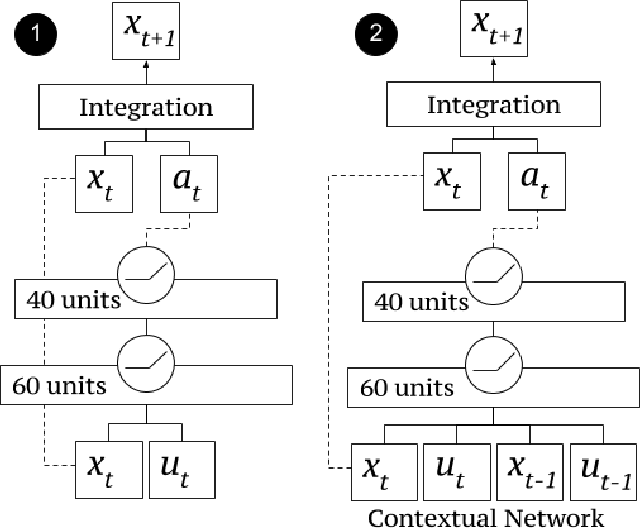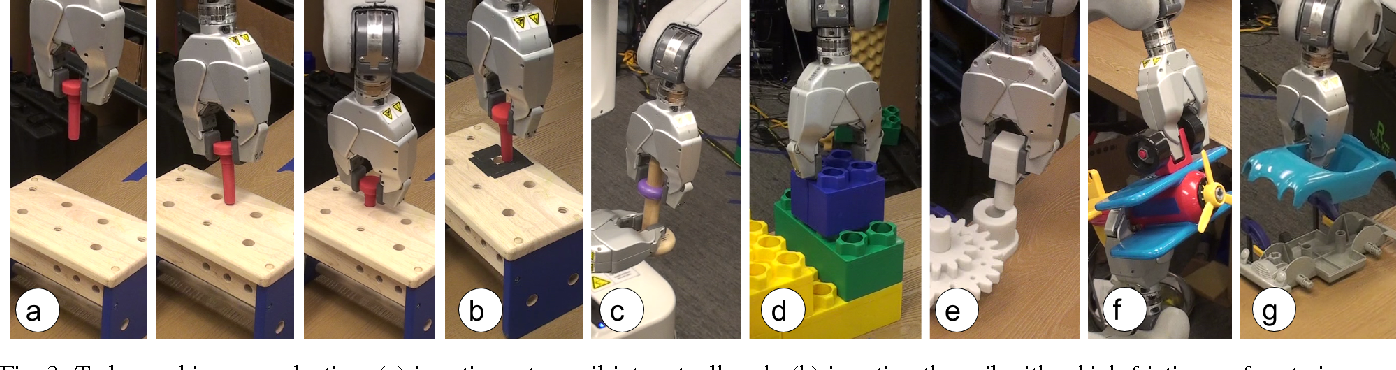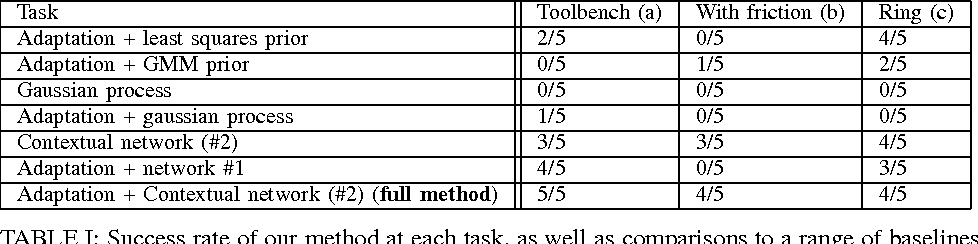One of the key challenges in applying reinforcement learning to complex robotic control tasks is the need to gather large amounts of experience in order to find an effective policy for the task at hand. Model-based reinforcement learning can achieve good sample efficiency, but requires the ability to learn a model of the dynamics that is good enough to learn an effective policy. In this work, we develop a model-based reinforcement learning algorithm that combines prior knowledge from previous tasks with online adaptation of the dynamics model. These two ingredients enable highly sample-efficient learning even in regimes where estimating the true dynamics is very difficult, since the online model adaptation allows the method to locally compensate for unmodeled variation in the dynamics. We encode the prior experience into a neural network dynamics model, adapt it online by progressively refitting a local linear model of the dynamics, and use model predictive control to plan under these dynamics. Our experimental results show that this approach can be used to solve a variety of complex robotic manipulation tasks in just a single attempt, using prior data from other manipulation behaviors.

Click to Read Paper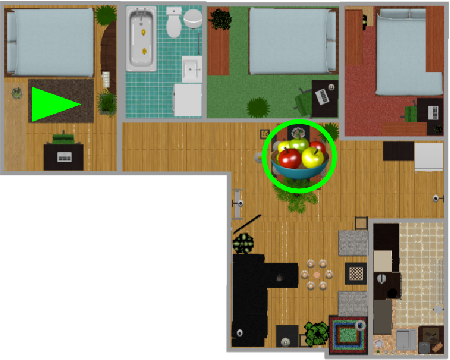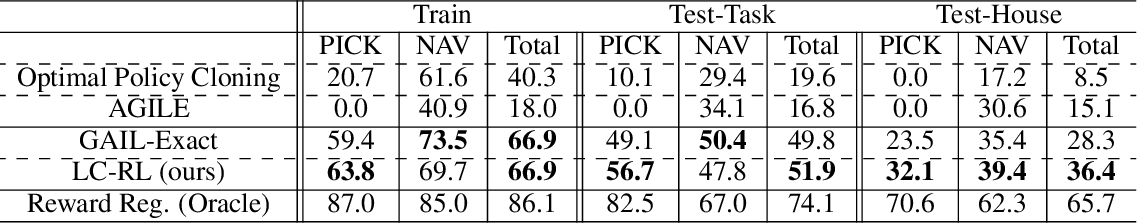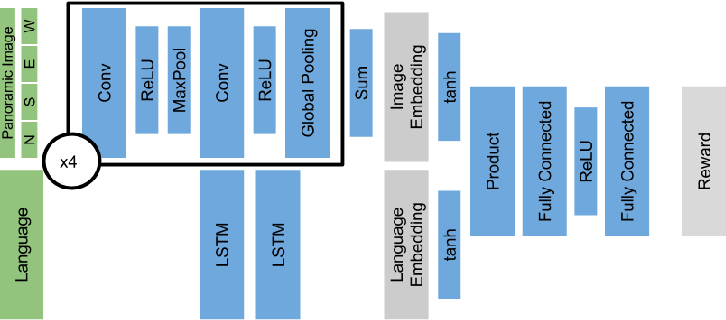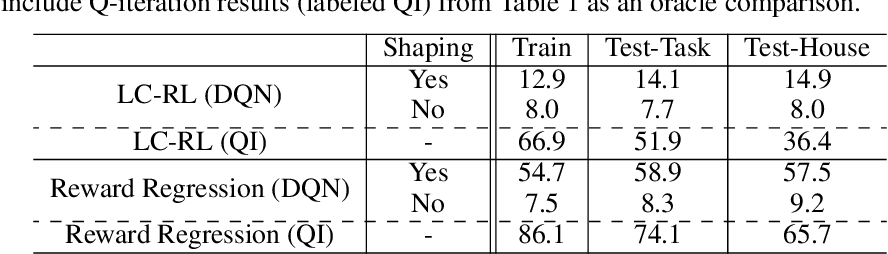Reinforcement learning is a promising framework for solving control problems, but its use in practical situations is hampered by the fact that reward functions are often difficult to engineer. Specifying goals and tasks for autonomous machines, such as robots, is a significant challenge: conventionally, reward functions and goal states have been used to communicate objectives. But people can communicate objectives to each other simply by describing or demonstrating them. How can we build learning algorithms that will allow us to tell machines what we want them to do? In this work, we investigate the problem of grounding language commands as reward functions using inverse reinforcement learning, and argue that language-conditioned rewards are more transferable than language-conditioned policies to new environments. We propose language-conditioned reward learning (LC-RL), which grounds language commands as a reward function represented by a deep neural network. We demonstrate that our model learns rewards that transfer to novel tasks and environments on realistic, high-dimensional visual environments with natural language commands, whereas directly learning a language-conditioned policy leads to poor performance.

Click to Read Paper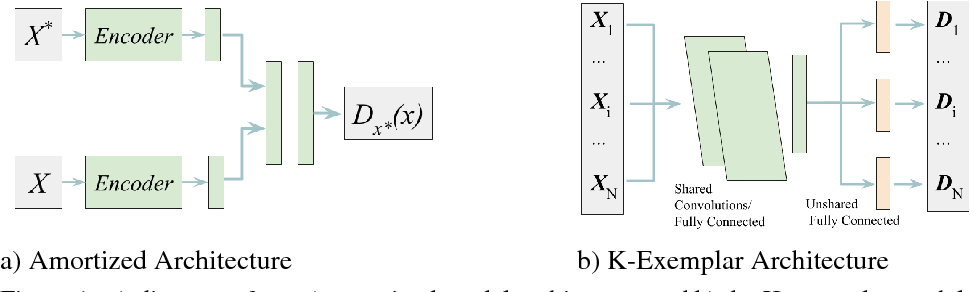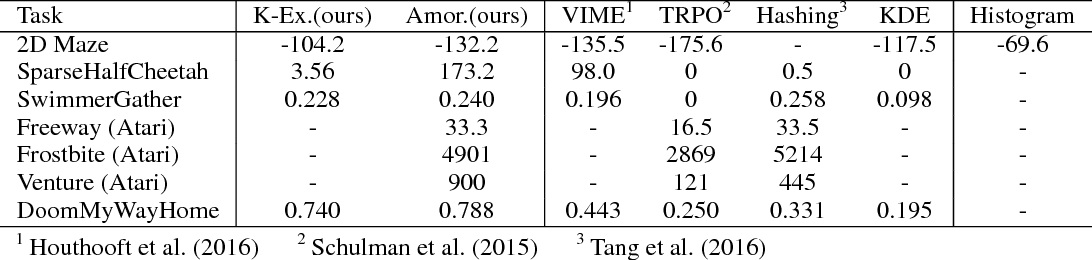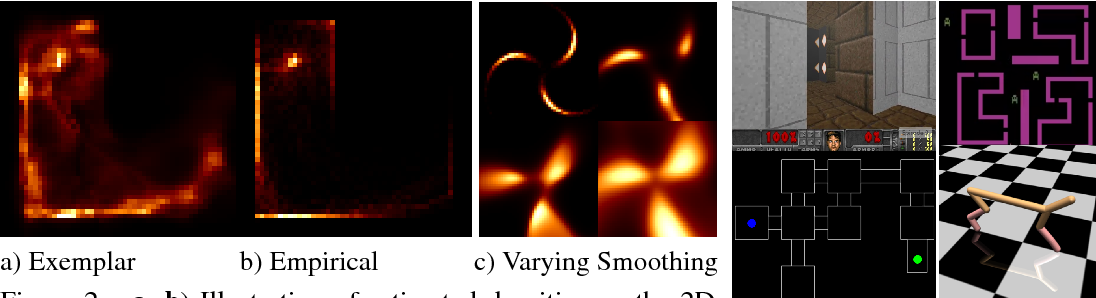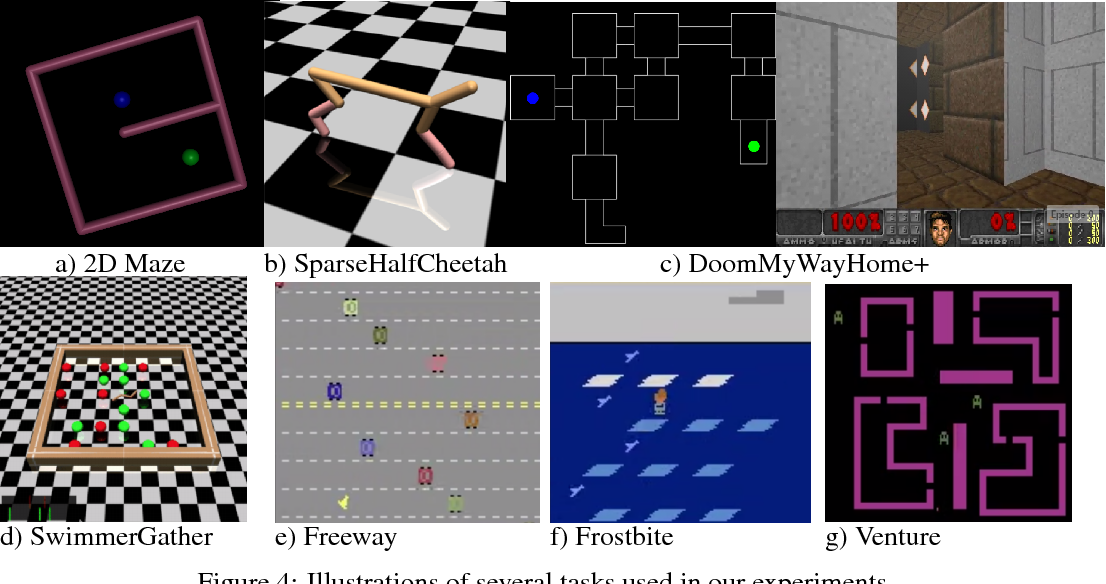Deep reinforcement learning algorithms have been shown to learn complex tasks using highly general policy classes. However, sparse reward problems remain a significant challenge. Exploration methods based on novelty detection have been particularly successful in such settings but typically require generative or predictive models of the observations, which can be difficult to train when the observations are very high-dimensional and complex, as in the case of raw images. We propose a novelty detection algorithm for exploration that is based entirely on discriminatively trained exemplar models, where classifiers are trained to discriminate each visited state against all others. Intuitively, novel states are easier to distinguish against other states seen during training. We show that this kind of discriminative modeling corresponds to implicit density estimation, and that it can be combined with count-based exploration to produce competitive results on a range of popular benchmark tasks, including state-of-the-art results on challenging egocentric observations in the vizDoom benchmark.

Click to Read Paper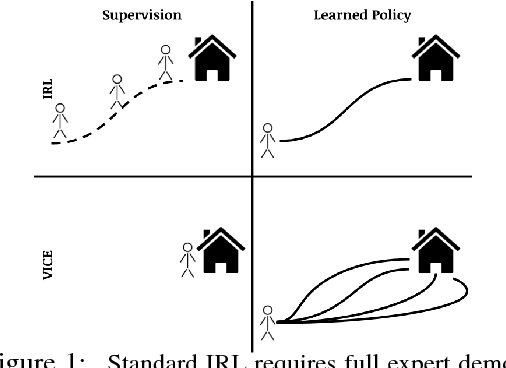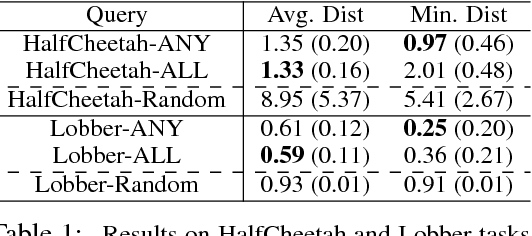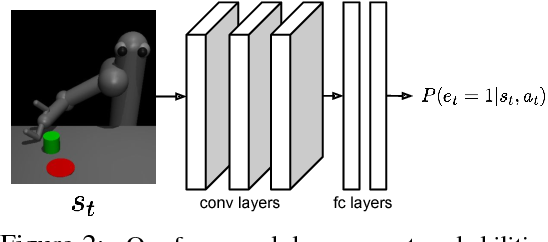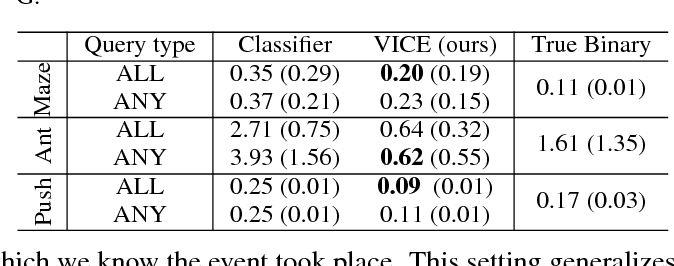The design of a reward function often poses a major practical challenge to real-world applications of reinforcement learning. Approaches such as inverse reinforcement learning attempt to overcome this challenge, but require expert demonstrations, which can be difficult or expensive to obtain in practice. We propose variational inverse control with events (VICE), which generalizes inverse reinforcement learning methods to cases where full demonstrations are not needed, such as when only samples of desired goal states are available. Our method is grounded in an alternative perspective on control and reinforcement learning, where an agent's goal is to maximize the probability that one or more events will happen at some point in the future, rather than maximizing cumulative rewards. We demonstrate the effectiveness of our methods on continuous control tasks, with a focus on high-dimensional observations like images where rewards are hard or even impossible to specify.

* First two authors contributed equally. Website: https://sites.google.com/view/inverse-event
Click to Read Paper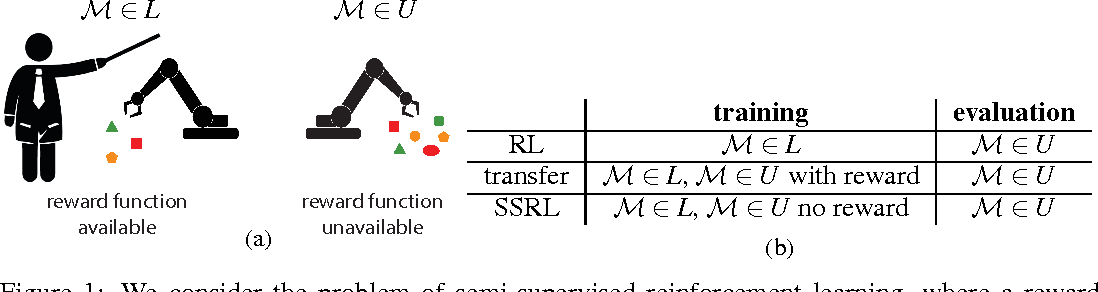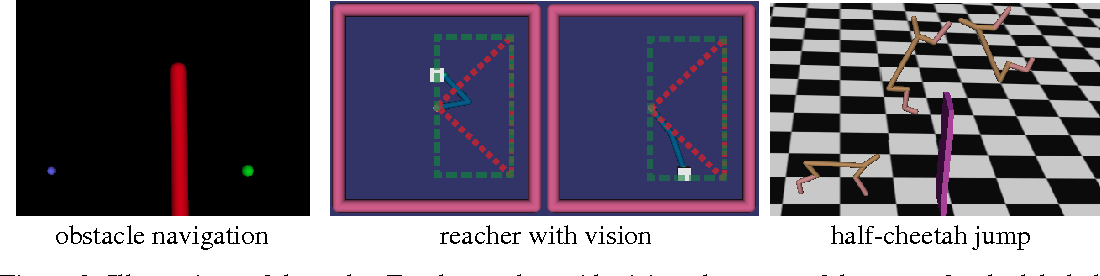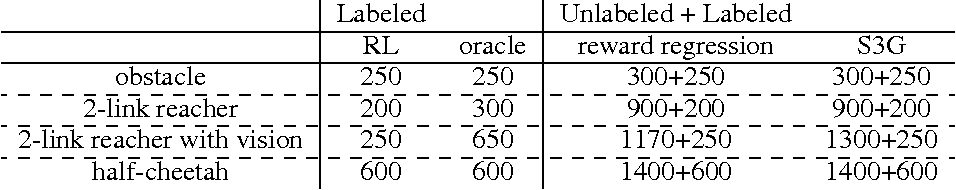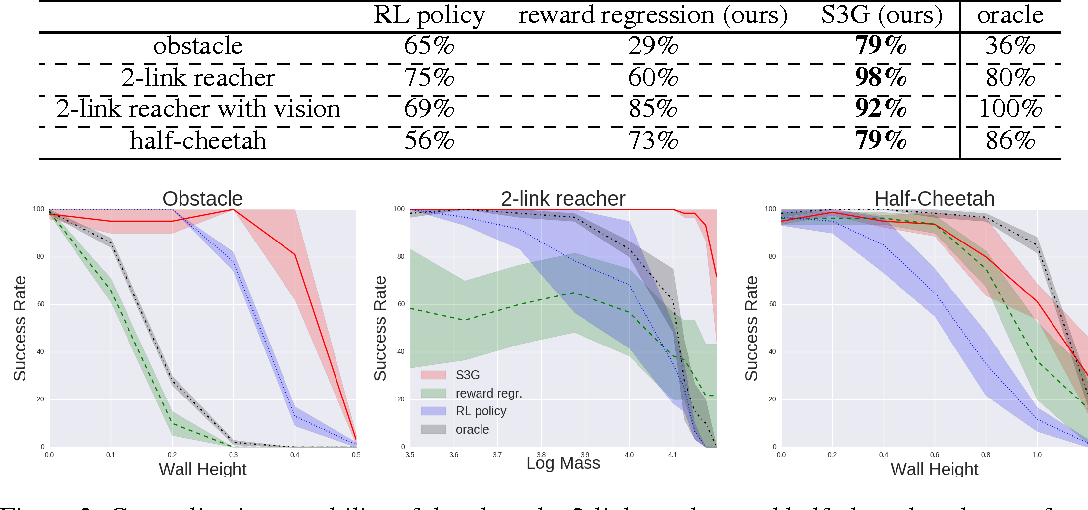Deep reinforcement learning (RL) can acquire complex behaviors from low-level inputs, such as images. However, real-world applications of such methods require generalizing to the vast variability of the real world. Deep networks are known to achieve remarkable generalization when provided with massive amounts of labeled data, but can we provide this breadth of experience to an RL agent, such as a robot? The robot might continuously learn as it explores the world around it, even while deployed. However, this learning requires access to a reward function, which is often hard to measure in real-world domains, where the reward could depend on, for example, unknown positions of objects or the emotional state of the user. Conversely, it is often quite practical to provide the agent with reward functions in a limited set of situations, such as when a human supervisor is present or in a controlled setting. Can we make use of this limited supervision, and still benefit from the breadth of experience an agent might collect on its own? In this paper, we formalize this problem as semisupervised reinforcement learning, where the reward function can only be evaluated in a set of "labeled" MDPs, and the agent must generalize its behavior to the wide range of states it might encounter in a set of "unlabeled" MDPs, by using experience from both settings. Our proposed method infers the task objective in the unlabeled MDPs through an algorithm that resembles inverse RL, using the agent's own prior experience in the labeled MDPs as a kind of demonstration of optimal behavior. We evaluate our method on challenging tasks that require control directly from images, and show that our approach can improve the generalization of a learned deep neural network policy by using experience for which no reward function is available. We also show that our method outperforms direct supervised learning of the reward.

* ICLR 2017
Click to Read Paper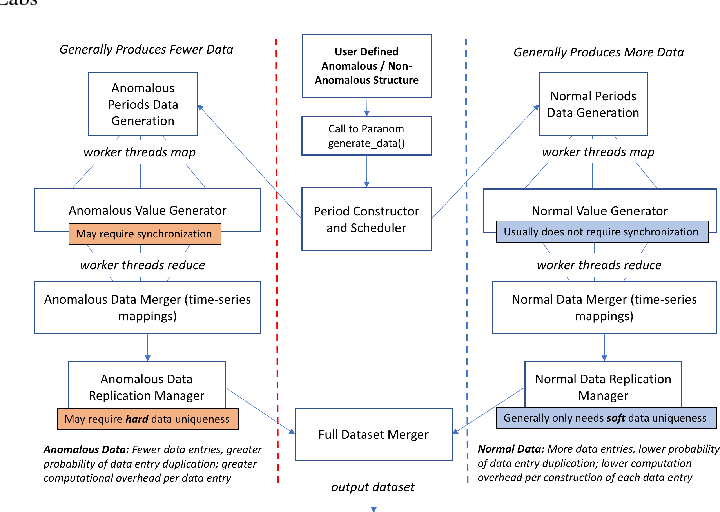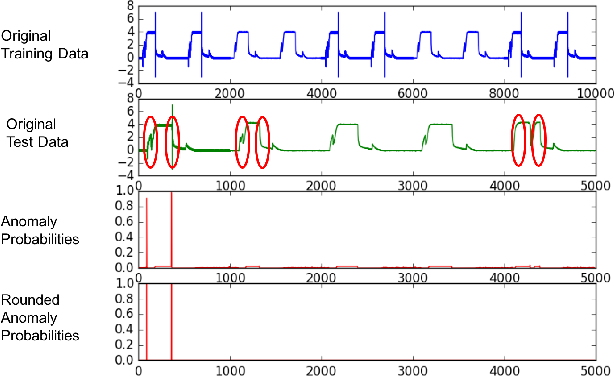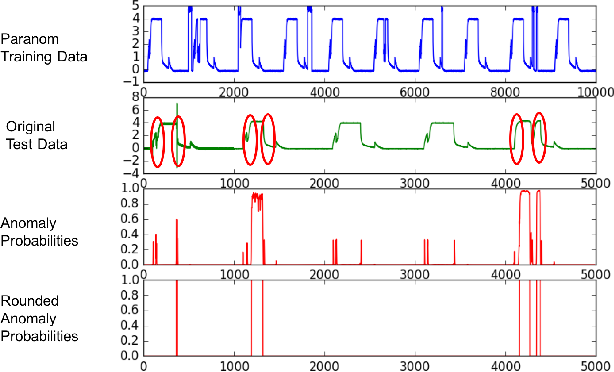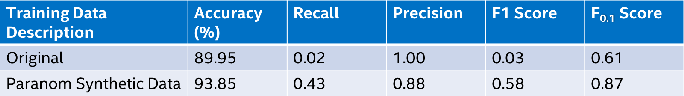In this paper, we present Paranom, a parallel anomaly dataset generator. We discuss its design and provide brief experimental results demonstrating its usefulness in improving the classification correctness of LSTM-AD, a state-of-the-art anomaly detection model.

Click to Read Paper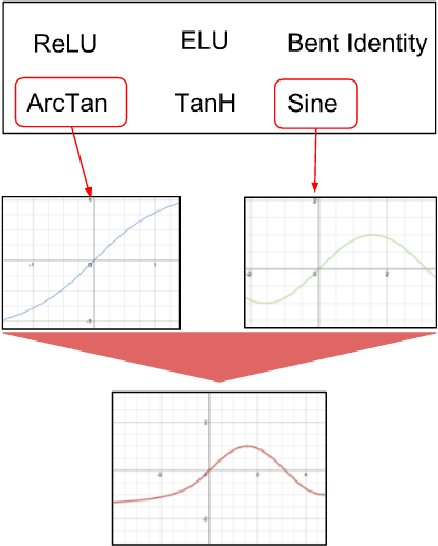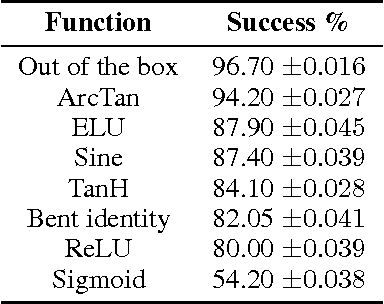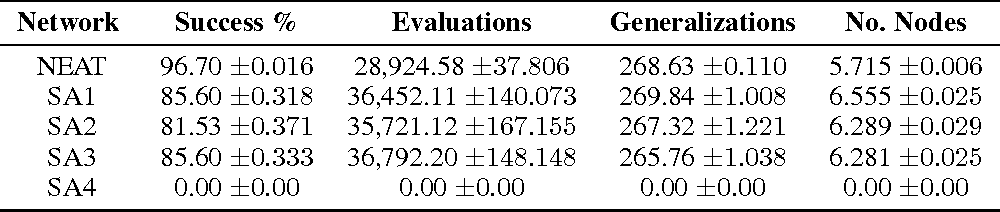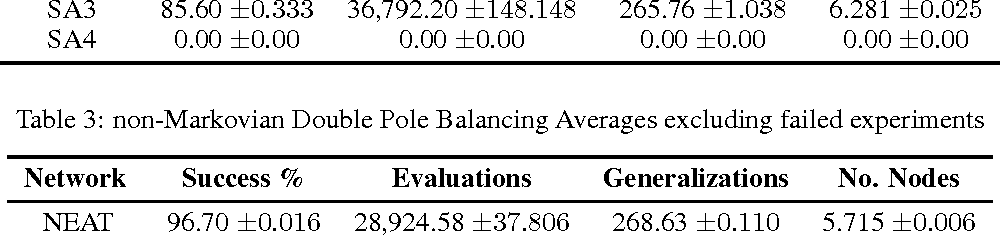In the neuroevolution literature, research has primarily focused on evolving the number of nodes, connections, and weights in artificial neural networks. Few attempts have been made to evolve activation functions. Research in evolving activation functions has mainly focused on evolving function parameters, and developing heterogeneous networks by selecting from a fixed pool of activation functions. This paper introduces a novel technique for evolving heterogeneous artificial neural networks through combinatorially generating piecewise activation functions to enhance expressive power. I demonstrate this technique on NeuroEvolution of Augmenting Topologies using ArcTan and Sigmoid, and show that it outperforms the original algorithm on non-Markovian double pole balancing. This technique expands the landscape of unconventional activation functions by demonstrating that they are competitive with canonical choices, and introduces a purview for further exploration of automatic model selection for artificial neural networks.

Click to Read Paper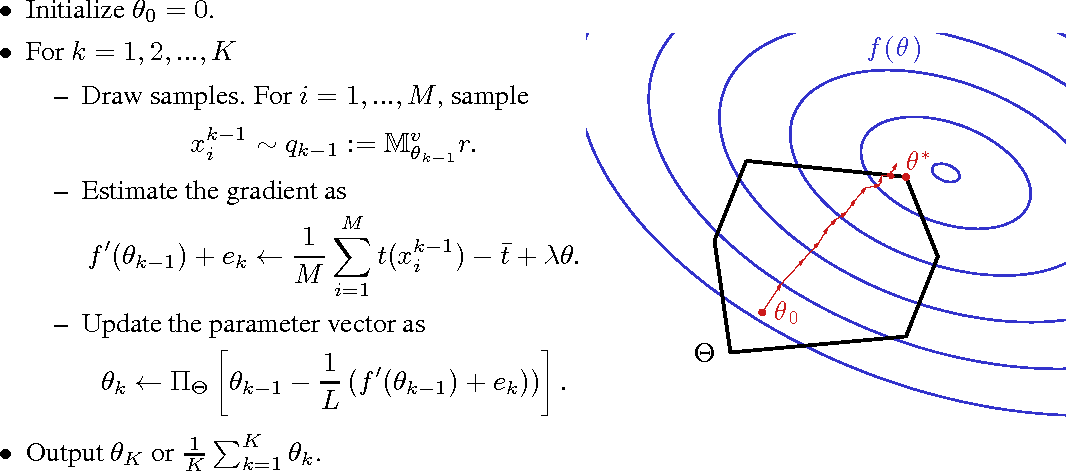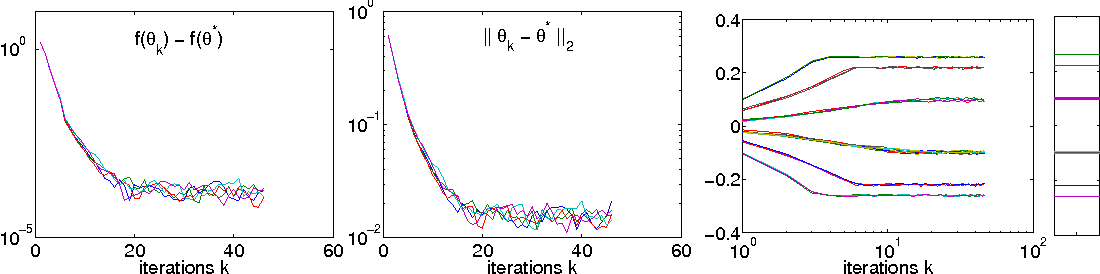Inference is typically intractable in high-treewidth undirected graphical models, making maximum likelihood learning a challenge. One way to overcome this is to restrict parameters to a tractable set, most typically the set of tree-structured parameters. This paper explores an alternative notion of a tractable set, namely a set of "fast-mixing parameters" where Markov chain Monte Carlo (MCMC) inference can be guaranteed to quickly converge to the stationary distribution. While it is common in practice to approximate the likelihood gradient using samples obtained from MCMC, such procedures lack theoretical guarantees. This paper proves that for any exponential family with bounded sufficient statistics, (not just graphical models) when parameters are constrained to a fast-mixing set, gradient descent with gradients approximated by sampling will approximate the maximum likelihood solution inside the set with high-probability. When unregularized, to find a solution epsilon-accurate in log-likelihood requires a total amount of effort cubic in 1/epsilon, disregarding logarithmic factors. When ridge-regularized, strong convexity allows a solution epsilon-accurate in parameter distance with effort quadratic in 1/epsilon. Both of these provide of a fully-polynomial time randomized approximation scheme.

* Advances in Neural Information Processing Systems 2015
Click to Read Paper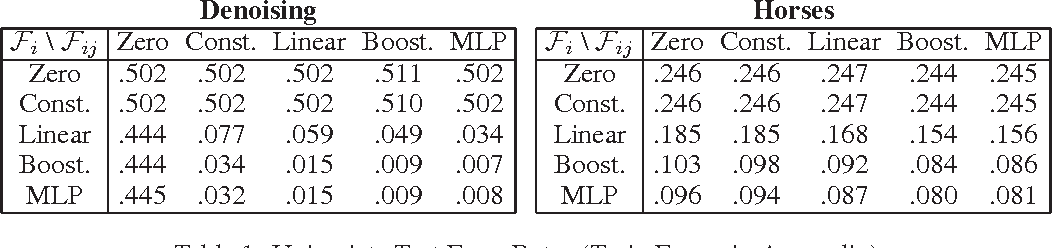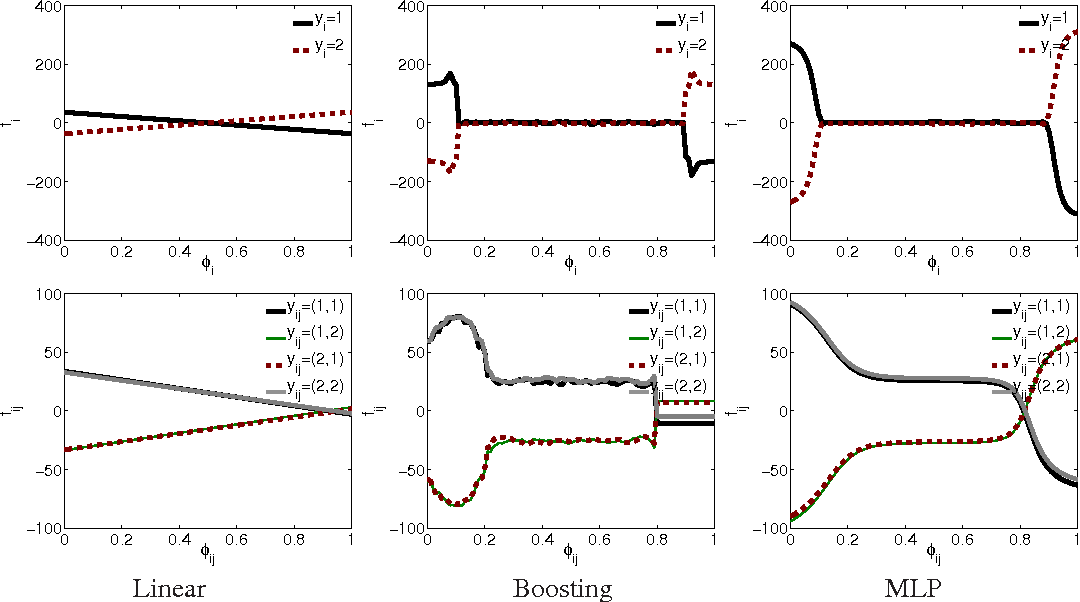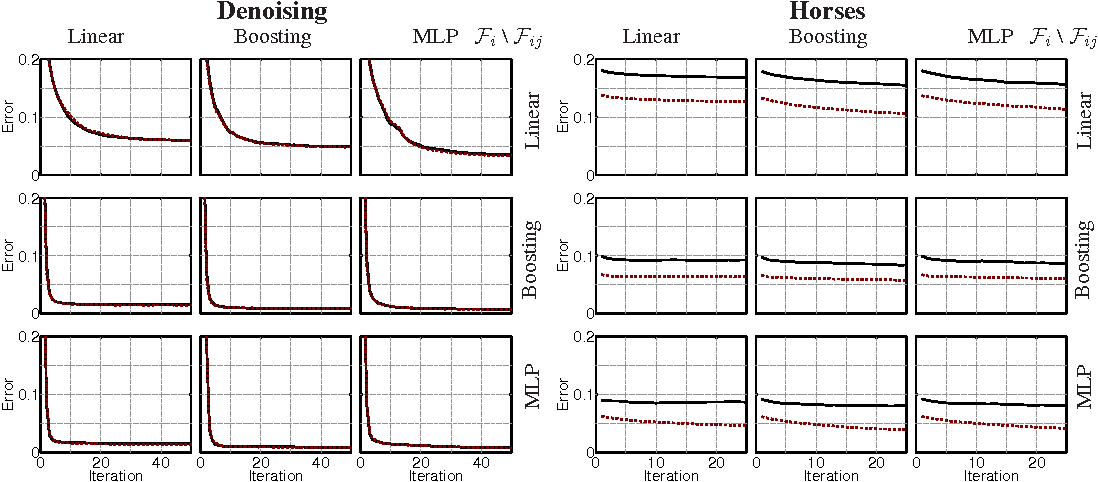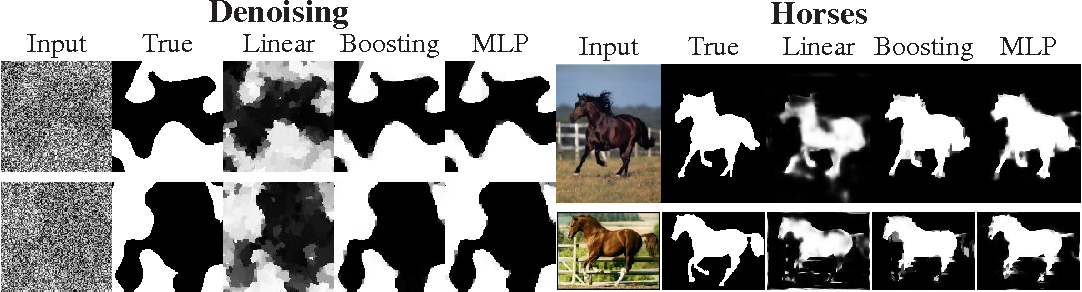A successful approach to structured learning is to write the learning objective as a joint function of linear parameters and inference messages, and iterate between updates to each. This paper observes that if the inference problem is "smoothed" through the addition of entropy terms, for fixed messages, the learning objective reduces to a traditional (non-structured) logistic regression problem with respect to parameters. In these logistic regression problems, each training example has a bias term determined by the current set of messages. Based on this insight, the structured energy function can be extended from linear factors to any function class where an "oracle" exists to minimize a logistic loss.

* Advances in Neural Information Processing Systems 2013
Click to Read Paper
Graphical models trained using maximum likelihood are a common tool for probabilistic inference of marginal distributions. However, this approach suffers difficulties when either the inference process or the model is approximate. In this paper, the inference process is first defined to be the minimization of a convex function, inspired by free energy approximations. Learning is then done directly in terms of the performance of the inference process at univariate marginal prediction. The main novelty is that this is a direct minimization of emperical risk, where the risk measures the accuracy of predicted marginals.

* Appears in Proceedings of the Twenty-Fourth Conference on Uncertainty in Artificial Intelligence (UAI2008)
Click to Read Paper
High-dimensional PDEs have been a longstanding computational challenge. We propose to solve high-dimensional PDEs by approximating the solution with a deep neural network which is trained to satisfy the differential operator, initial condition, and boundary conditions. Our algorithm is meshfree, which is key since meshes become infeasible in higher dimensions. Instead of forming a mesh, the neural network is trained on batches of randomly sampled time and space points. The algorithm is tested on a class of high-dimensional free boundary PDEs, which we are able to accurately solve in up to $200$ dimensions. The algorithm is also tested on a high-dimensional Hamilton-Jacobi-Bellman PDE and Burgers' equation. The deep learning algorithm approximates the general solution to the Burgers' equation for a continuum of different boundary conditions and physical conditions (which can be viewed as a high-dimensional space). We call the algorithm a "Deep Galerkin Method (DGM)" since it is similar in spirit to Galerkin methods, with the solution approximated by a neural network instead of a linear combination of basis functions. In addition, we prove a theorem regarding the approximation power of neural networks for a class of quasilinear parabolic PDEs.

* Deep learning, machine learning, partial differential equations
Click to Read Paper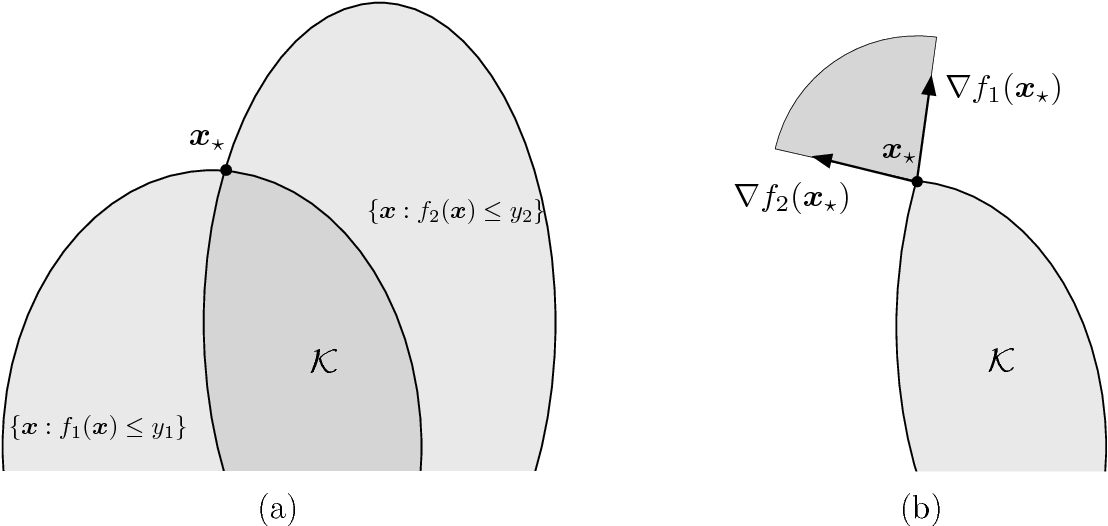We consider the question of estimating a solution to a system of equations that involve convex nonlinearities, a problem that is common in machine learning and signal processing. Because of these nonlinearities, conventional estimators based on empirical risk minimization generally involve solving a non-convex optimization program. We propose anchored regression, a new approach based on convex programming that amounts to maximizing a linear functional (perhaps augmented by a regularizer) over a convex set. The proposed convex program is formulated in the natural space of the problem, and avoids the introduction of auxiliary variables, making it computationally favorable. Working in the native space also provides great flexibility as structural priors (e.g., sparsity) can be seamlessly incorporated. For our analysis, we model the equations as being drawn from a fixed set according to a probability law. Our main results provide guarantees on the accuracy of the estimator in terms of the number of equations we are solving, the amount of noise present, a measure of statistical complexity of the random equations, and the geometry of the regularizer at the true solution. We also provide recipes for constructing the anchor vector (that determines the linear functional to maximize) directly from the observed data.

Click to Read Paper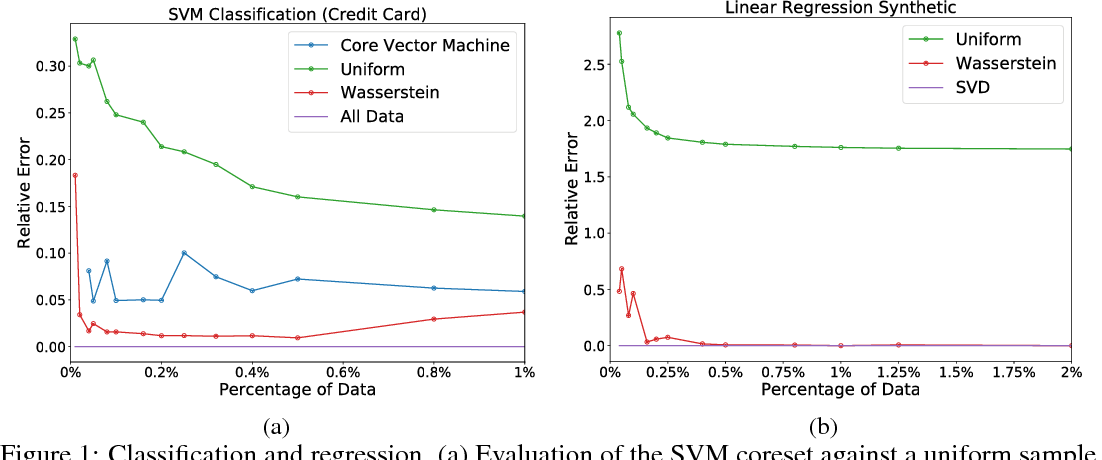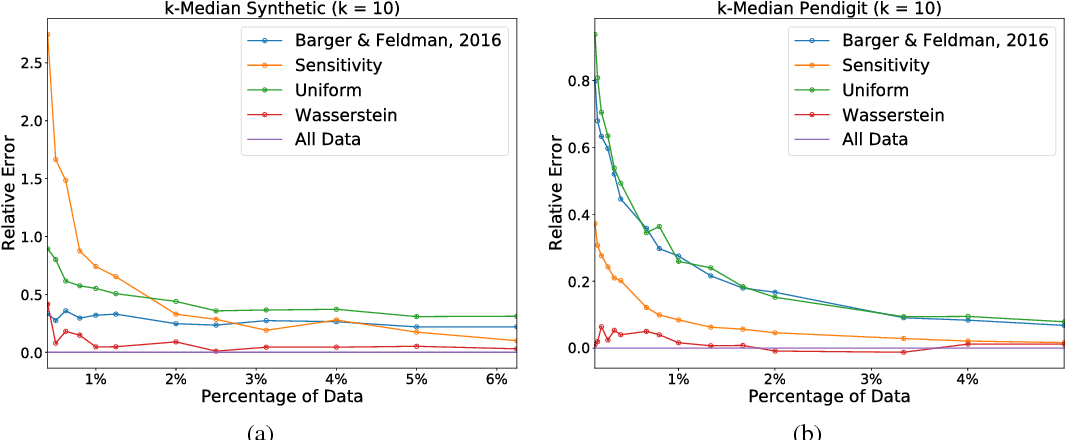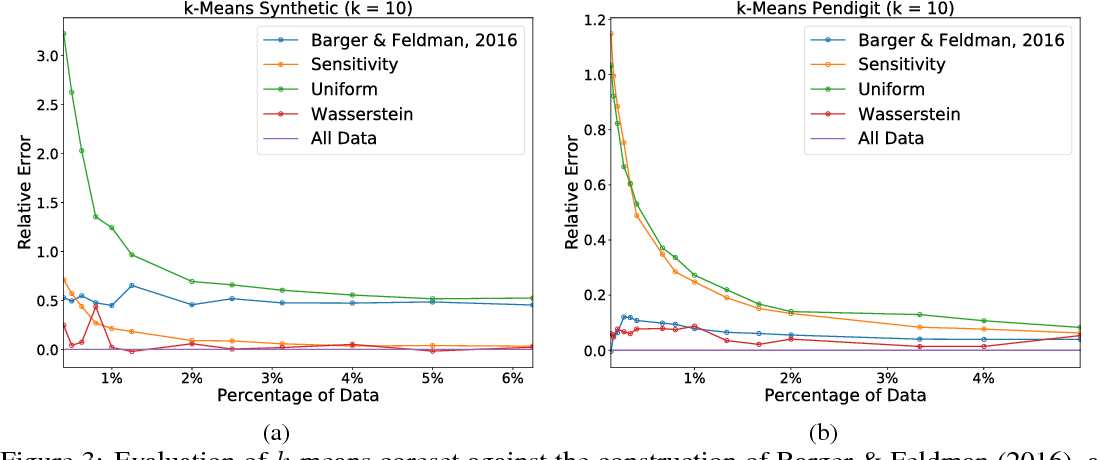Sparsification is becoming more and more relevant with the proliferation of huge data sets. Coresets are a principled way to construct representative weighted subsets of a data set that have matching performance with the full data set for specific problems. However, coreset language neglects the nature of the underlying data distribution, which is often continuous. In this paper, we address this oversight by introducing a notion of measure coresets that generalizes coreset language to arbitrary probability measures. Our definition reveals a surprising connection to optimal transport theory which we leverage to design a coreset for problems with Lipschitz costs. We validate our construction on support vector machine (SVM) training, k-means clustering, k-median clustering, and linear regression and show that we are competitive with previous coreset constructions.

Click to Read Paper
Stochastic gradient descent in continuous time (SGDCT) provides a computationally efficient method for the statistical learning of continuous-time models, which are widely used in science, engineering, and finance. The SGDCT algorithm follows a (noisy) descent direction along a continuous stream of data. The parameter updates occur in continuous time and satisfy a stochastic differential equation. This paper analyzes the asymptotic convergence rate of the SGDCT algorithm by proving a central limit theorem for strongly convex objective functions and, under slightly stronger conditions, for non-convex objective functions as well. An L$^p$ convergence rate is also proven for the algorithm in the strongly convex case.

Click to Read Paper
Stochastic gradient descent in continuous time (SGDCT) provides a computationally efficient method for the statistical learning of continuous-time models, which are widely used in science, engineering, and finance. The SGDCT algorithm follows a (noisy) descent direction along a continuous stream of data. SGDCT performs an online parameter update in continuous time, with the parameter updates $\theta_t$ satisfying a stochastic differential equation. We prove that $\lim_{t \rightarrow \infty} \nabla \bar g(\theta_t) = 0$ where $\bar g$ is a natural objective function for the estimation of the continuous-time dynamics. The convergence proof leverages ergodicity by using an appropriate Poisson equation to help describe the evolution of the parameters for large times. SGDCT can also be used to solve continuous-time optimization problems, such as American options. For certain continuous-time problems, SGDCT has some promising advantages compared to a traditional stochastic gradient descent algorithm. As an example application, SGDCT is combined with a deep neural network to price high-dimensional American options (up to 100 dimensions).

Click to Read Paper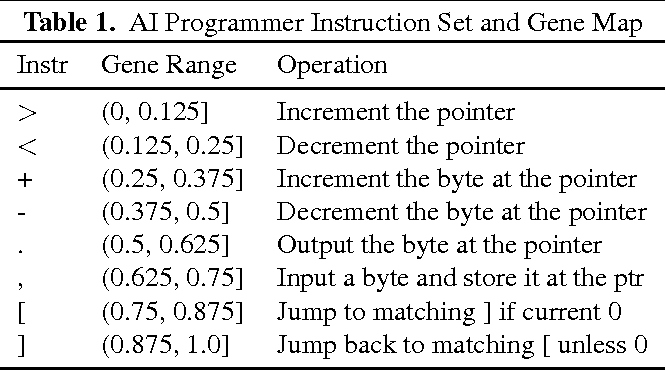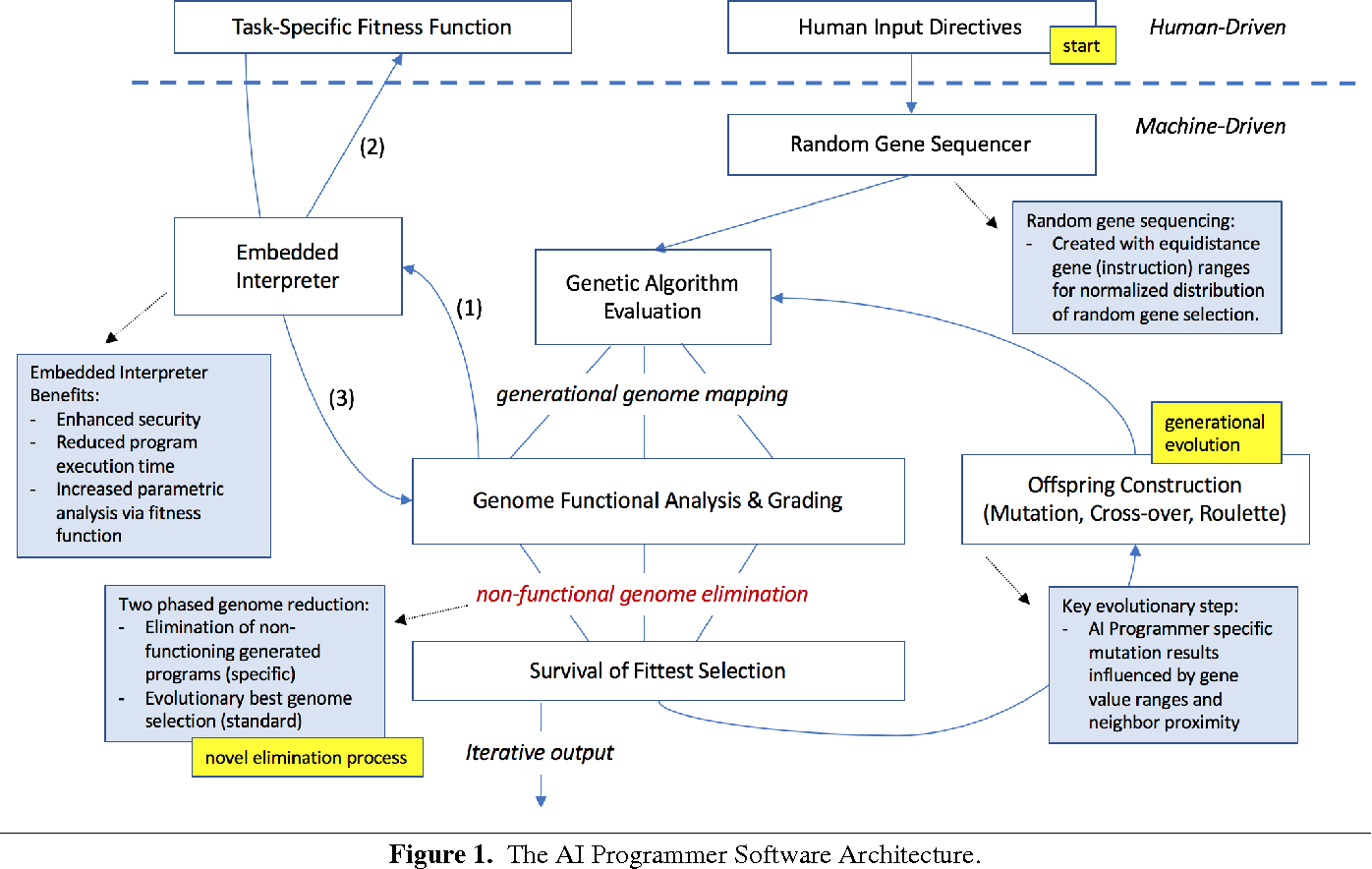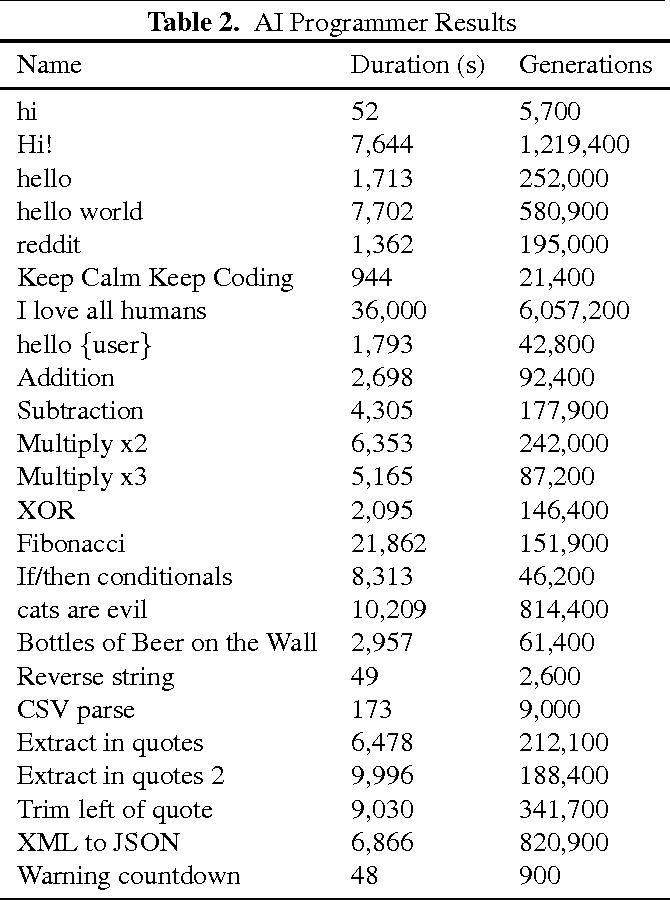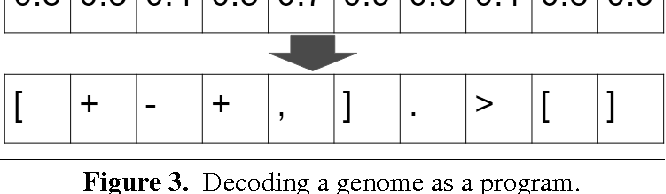In this paper, we present the first-of-its-kind machine learning (ML) system, called AI Programmer, that can automatically generate full software programs requiring only minimal human guidance. At its core, AI Programmer uses genetic algorithms (GA) coupled with a tightly constrained programming language that minimizes the overhead of its ML search space. Part of AI Programmer's novelty stems from (i) its unique system design, including an embedded, hand-crafted interpreter for efficiency and security and (ii) its augmentation of GAs to include instruction-gene randomization bindings and programming language-specific genome construction and elimination techniques. We provide a detailed examination of AI Programmer's system design, several examples detailing how the system works, and experimental data demonstrating its software generation capabilities and performance using only mainstream CPUs.

Click to Read Paper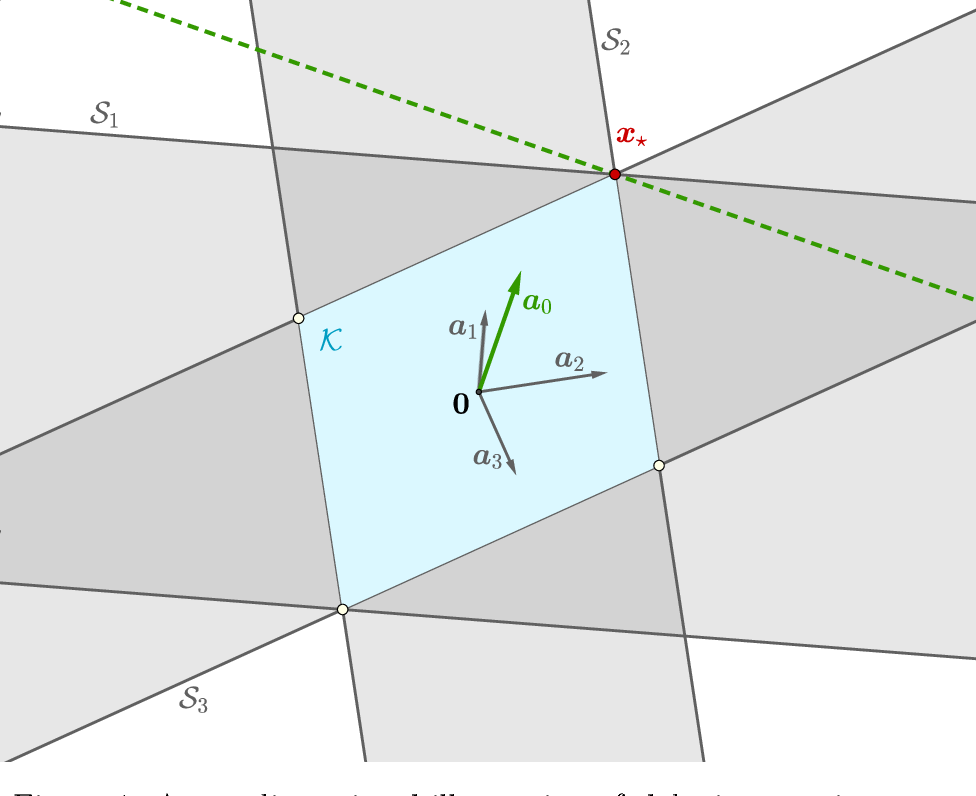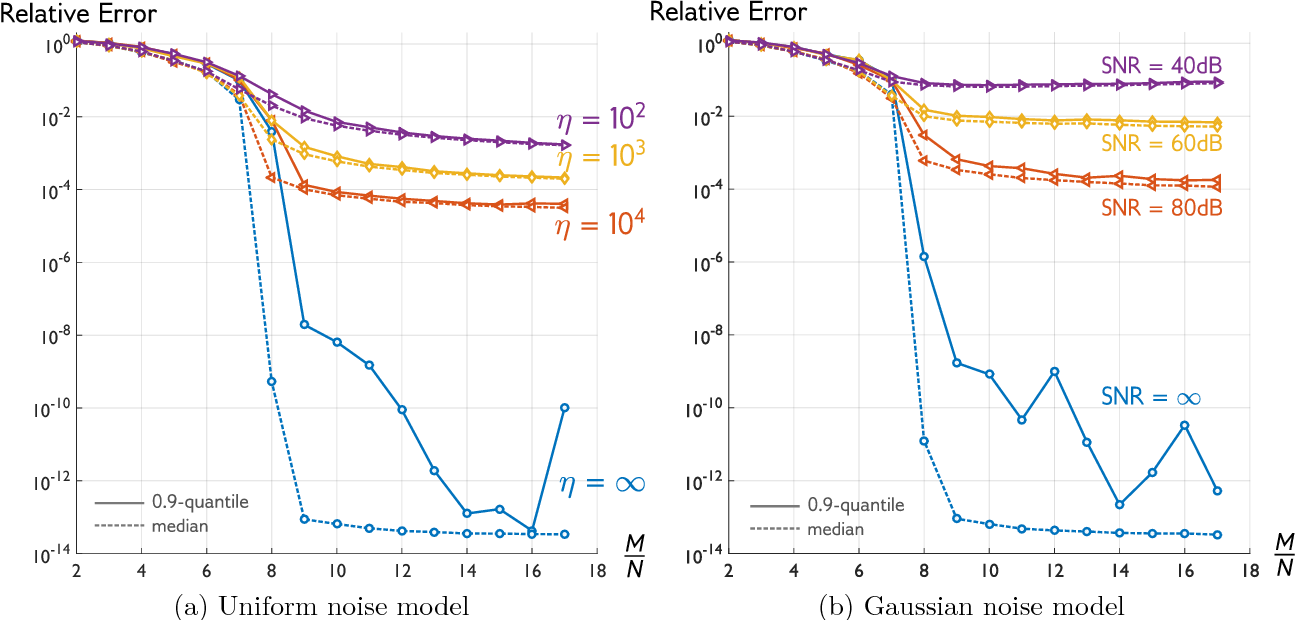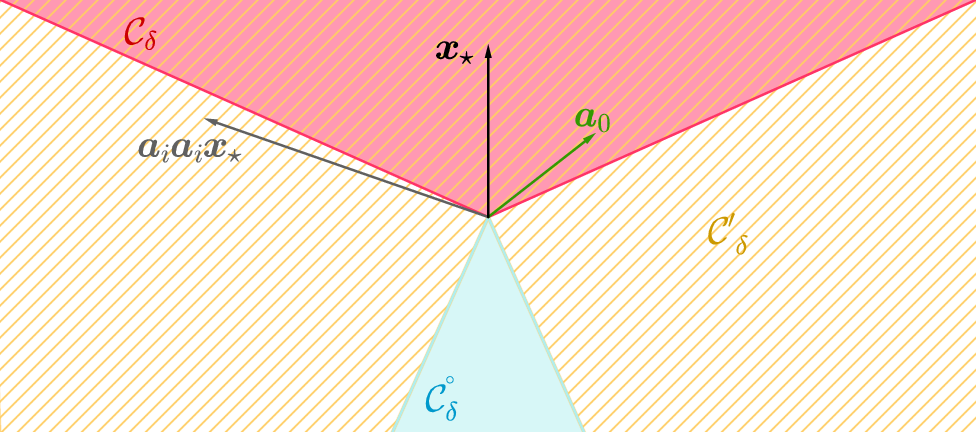We propose a flexible convex relaxation for the phase retrieval problem that operates in the natural domain of the signal. Therefore, we avoid the prohibitive computational cost associated with "lifting" and semidefinite programming (SDP) in methods such as PhaseLift and compete with recently developed non-convex techniques for phase retrieval. We relax the quadratic equations for phaseless measurements to inequality constraints each of which representing a symmetric "slab". Through a simple convex program, our proposed estimator finds an extreme point of the intersection of these slabs that is best aligned with a given anchor vector. We characterize geometric conditions that certify success of the proposed estimator. Furthermore, using classic results in statistical learning theory, we show that for random measurements the geometric certificates hold with high probability at an optimal sample complexity. Phase transition of our estimator is evaluated through simulations. Our numerical experiments also suggest that the proposed method can solve phase retrieval problems with coded diffraction measurements as well.

* Accepted in AISTATS 2017. Extended the discussion of related work and added a few more references. Clarified some of the statements and notations
Click to Read Paper
We examine the effect of clamping variables for approximate inference in undirected graphical models with pairwise relationships and discrete variables. For any number of variable labels, we demonstrate that clamping and summing approximate sub-partition functions can lead only to a decrease in the partition function estimate for TRW, and an increase for the naive mean field method, in each case guaranteeing an improvement in the approximation and bound. We next focus on binary variables, add the Bethe approximation to consideration and examine ways to choose good variables to clamp, introducing new methods. We show the importance of identifying highly frustrated cycles, and of checking the singleton entropy of a variable. We explore the value of our methods by empirical analysis and draw lessons to guide practitioners.

Click to Read Paper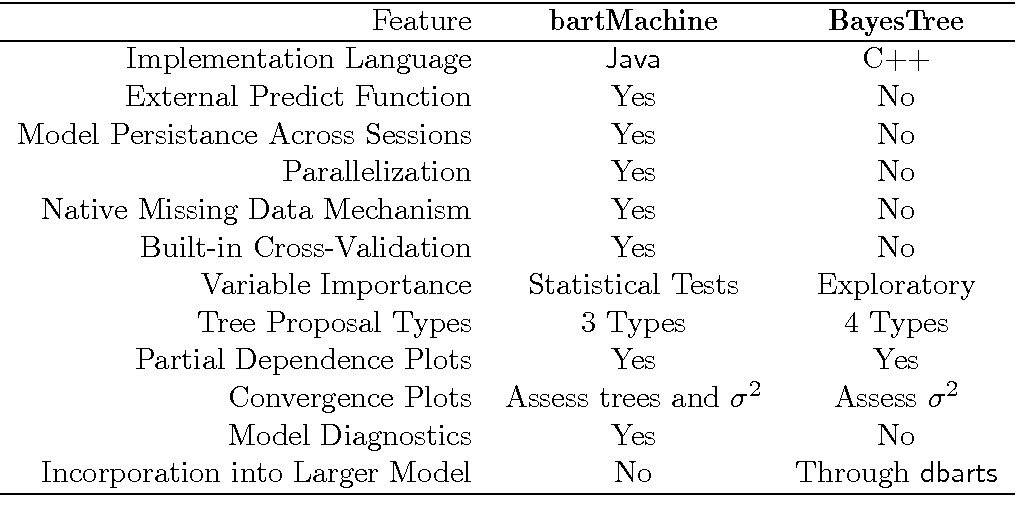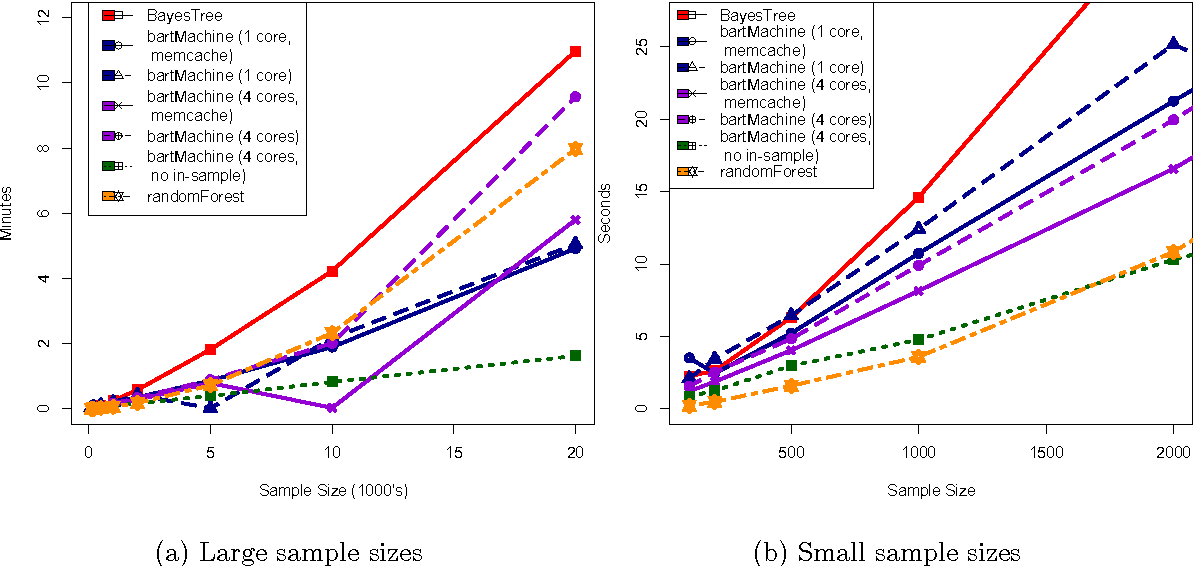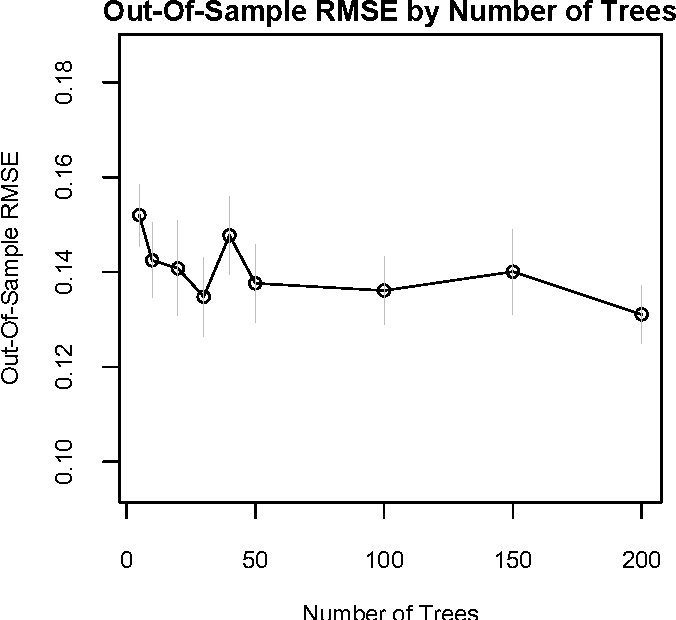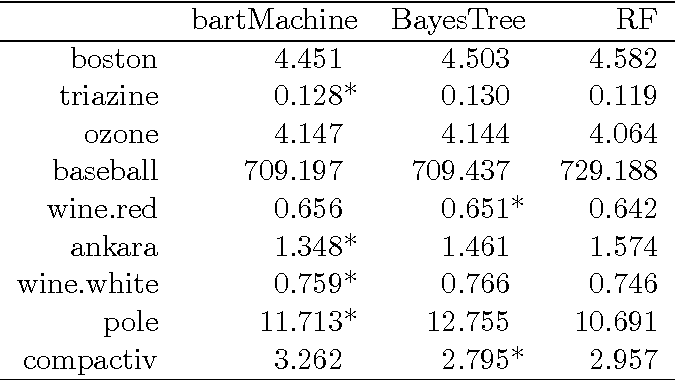We present a new package in R implementing Bayesian additive regression trees (BART). The package introduces many new features for data analysis using BART such as variable selection, interaction detection, model diagnostic plots, incorporation of missing data and the ability to save trees for future prediction. It is significantly faster than the current R implementation, parallelized, and capable of handling both large sample sizes and high-dimensional data.

* 39 pages, 13 figures, 4 tables, 2 appendices
Click to Read Paper